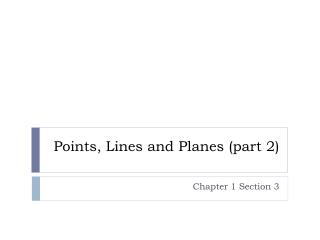DownloadDownload PresentationPoints, Lines and Planes (part 2)

# Points, Lines and Planes (part 2)

Download Presentation## Points, Lines and Planes (part 2)

- - - - - - - - - - - - - - - - - - - - - - - - - - - E N D - - - - - - - - - - - - - - - - - - - - - - - - - - -
##### Presentation Transcript

1. Points, Lines and Planes (part 2) Chapter 1 Section 3

2. postulate or axiom: a statement accepted as fact (no proof needed) postulates and axioms (and later theorems) are numbered in your book, but that is onlyfor the purpose of keeping the book organized. Know the postulate/axiom, NOT it’s number.

3. Postulate 1-1 • Through any two points there is exactly one line • In other words: two points determine a unique line

4. Postulate 1-2 • If two lines intersect, then they intersect in exactly one point. Graph: Graph:

5. Postulate 1-3 • If two planes intersect, then they intersect in exactly one line. • In other words: the intersection of two planes is a line. H What is the intersection of plane EFB and plane ABC (the bottom)? What is the intersection of plane BCG and plane HGC? What is the intersection of plane ABF and plane CGF? G F E C A B

6. Postulate 1-4 • Through any three noncollinear points there is exactly one plane. • In other words, three points determine a plane.

7. Classwork: p 20 # 17 – 37 Homework: Remainder of 1-3 worksheet